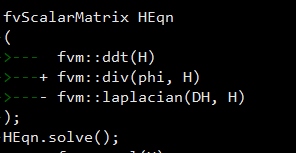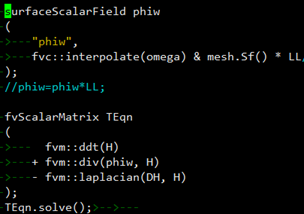# 新方程的预测步和修正步

• 想向各位大佬请教一下，在icofoam里面添加新方程$\frac{\partial H}{\partial t}+ \vec{u}\cdot \triangledown H=\varepsilon \triangledown ^{2}H$和$\frac{\partial H}{\partial t}+ \vec{w}\cdot \triangledown H=\varepsilon \triangledown ^{2}H$,其中$\varepsilon$是常数，H是一个标量函数。但是不知道为什么得到的H是只算了第二个方程的结果，一个方程相当于没有算。代码如下：• 在同一个时间步里面植入两个方程，需要把H.oldTime()重新赋值一下

• 谢谢东岳老师